Clustered Sparse Associative Memories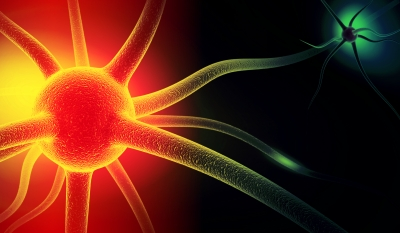Associative memories, unlike a traditional memory system which requires explicit addresses separated from its content, are devices mapping input-output patterns and retrieve information from its context directly. Given a partial or corrupt probe, associative memories retrieve the most relevant or similar ones among all previously stored messages. The Gripon-Berrou Neural Network is a recently proposed recurrent network with a partite cluster structure featuring iterative computing schemes. It can be used to implement associative memories, and we call this model Clustered Sparse Associative Memories (CSAM). Due to its close connection to a LDPC-like sparse binary coding, it is able to retrieve the information robustly and efficiently even under severe distortions.

In this project, we study various iterative methods to combat against partially erased probes and implement the network on GPUs, accelerating 900x without any loss of accuracy. We discover a formerly overlooked problem and propose heuristics to bypass the problem in a distributed manner. A new post-processing algorithm, related to the maximum clique problem, is also developed, which improves the successful retrieval rate significantly. Moreover, we extend GBNNs against corrupt probes, greatly expanding the network utility.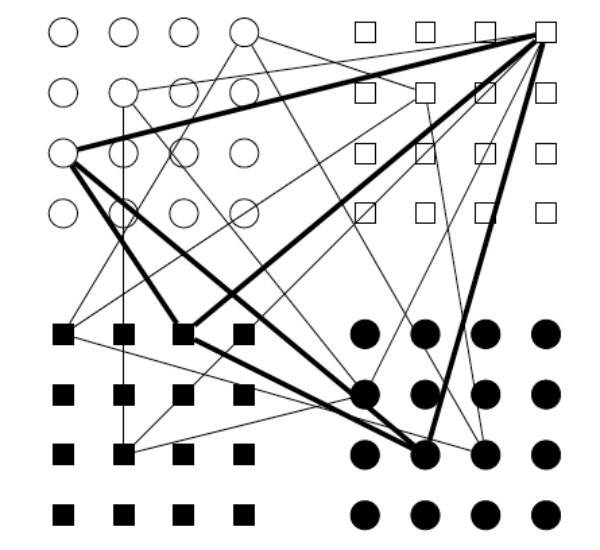The structure of a CSAM network is closely related to the stored messages. Consider a message of a \$C\$-symbol tuple, \$(m_1,m_2,\cdots,m_C)\$, with each symbol \$m_c\$ taking one value from a finite alphabet of size \$L\$, i.e., \$m_c=x_l,l=1,2,\cdots,L\$. A network of \$n=CL\$ neurons is used in this setup, with \$C\$ clusters corresponding to different symbols, each having \$L\$ neurons representing the value \$x_l\$. If \$m_c=x_l\$, the \$l\$th neuron in the \$c\$th cluster, neuron\$(c,l)\$, activates. Since a symbol can only take one value at a time, for a given message, in each cluster, there is only one single active neuron accordingly. The locations of the active neurons express a particular message.

The CSAM network is a binary valued neural network in the sense that the weights on connections between a pair of neurons can either exist (1) or not (0). After fixing a message, the corresponding active neurons and the connections in between form a clique (complete sub-graph). The graph on the right depicts a typical CSAM network with \$C=4\$ clusters and \$L=16\$ neurons each. We label ○ for cluster 1, ◻ for cluster 2, ◼ for cluster 3 and ● for cluster 4. We also number the neurons sequentially row by row from 1 to 16 in each cluster. Three messages are stored in the network, with the black clique indicates the message \$(x_9,x_4,x_3,x_{10})\$. To retrieve a message, a probe is given, either a partial message, e.g., \$(x_9,?,?,x_{10})\$, or a corrupt message, e.g., \$(x_9,x_3,x_3,x_{10})\$, and the network is queried which stored message is most similar to the probe.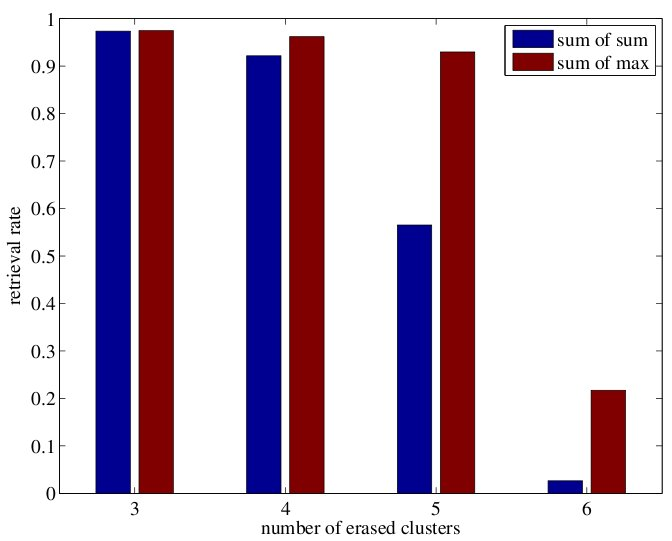In the retrieving stage, active neurons are assumed as energy sources, sending out signals along the edges recorded in the learning stage. Two basic iterative algorithms exist to retrieve a message. One is called SUM-OF-SUM, the other SUM-OF-MAX. Given a probe, the former counts individual signals and activate the neuron with the most signals in each cluster for the next iteration, whereas the latter counts clusterwise signals so that the signals from the same cluster do not sum up with only the neurons receiving signals from all clusters being kept active.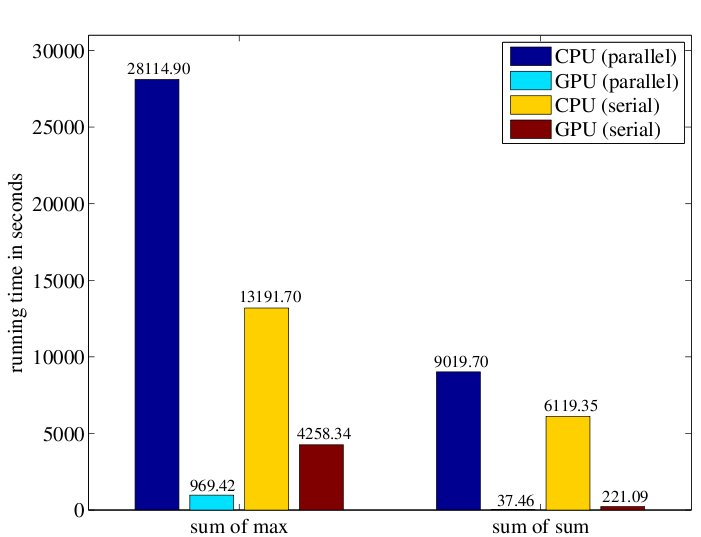In , we demonstrate a counter example showing that SUM-OF-SUM may oscillate, but we manage to prove the convergence of SUM-OF-MAX. This is one of the reasons we favour SUM-OF-MAX. Another important reason is that SUM-OF-SUM tends to accumulate decoding errors from iteration to iteration, deteriorating retrieval rate drastically, especially in heavily loaded networks or when the retrieving stage is challenging; see the top left plot.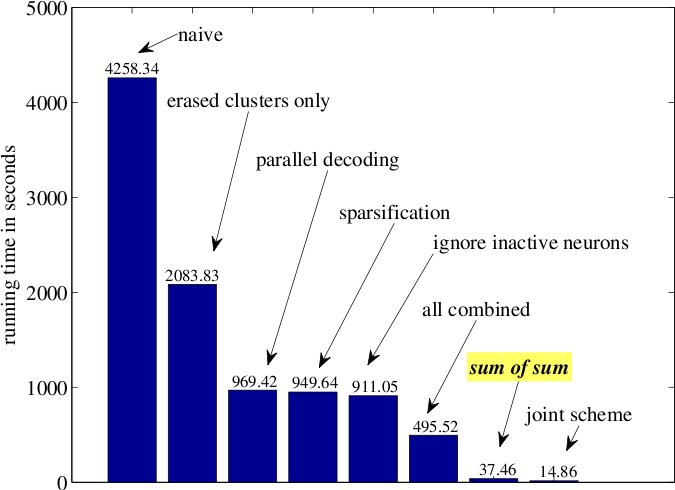We implement both retrieving algorithms on GPUs, bringing us exciting speedup compared with C++ libraries linked to LAPACK/BLAS using CPU; see the right plot. Since SUM-OF-SUM reduces to a matrix-vector multiplication, a highly optimized operation on GPUs, it runs orders of magnitude faster by default than the standard version of SUM-OF-MAX, which involves time consuming non-linear operators. We successfully break down the algorithm and accelerate SUM-OF-MAX using various techniques. Moreover, a joint scheme mixing both SUM-OF-SUM and SUM-OF-MAX is also developed. Compared with CPU implementations, an acceleration of 900x is witnessed, without sacrificing any retrieval rate; see bottom left plot.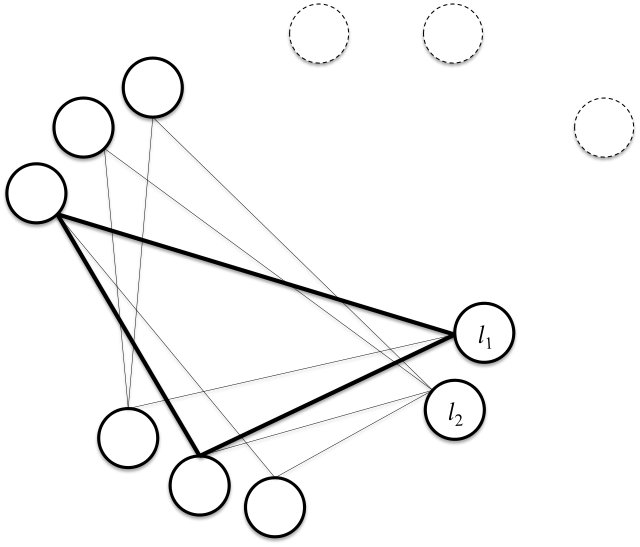In previous studies, SUM-OF-MAX is believed to converge to the ensemble of stored messages. However, there is an overlooked problem that the network converges to a bogus fixed point, where the ensemble of stored messages only comprises a small subset of the state. See the graph on the right, each dashed circle represents the only active neuron in some cluster, and they connect to all neurons in the bottom left corner. Three clusters of interest are at the corner with every neuron receiving signals from all clusters. Therefore, the network keeps all of them active when it converges. Unfortunately, only the black triangle is the desired clique worth preserving. Choosing an arbitrary neuron as the retrieved message, when the network reaches a bogus fixed point, undermining the performance severely. In , we propose six distributed heuristics, escaping from the bogus fixed point. Although the heuristics helps to increase the retrieval rate, none is guaranteed to find the desired clique.

To fix the problem directly and completely, a novel centralized post-processing algorithm is developed. It is based on finding a maximum clique in a graph, which is a famous NP-hard problem. However, we successfully adapt the algorithm to fully exploit CSAM's stringent nice C-partite structure, which not only brings us astonishing retrieval rate, but also beats all the heuristics in terms of processing time by a large margin.

The following plots are based on the famous USPS hand written digits, showing the numerical comparisons among our novel algorithm (Algorithm 2), the standard SUM-OF-MAX and the celebrated Willshaw associative memory model. One can easily notice the retrieval rates improvements both symbol-wise and message-wise.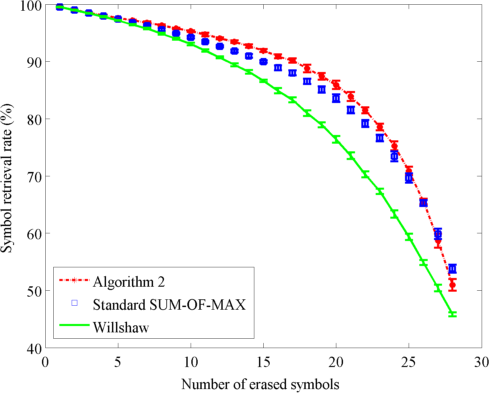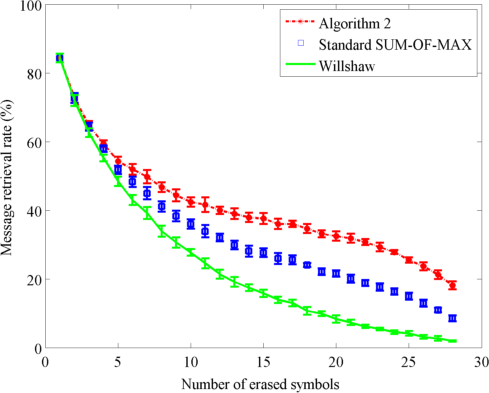To better visualize the advantage of our approach, instances of the digit images are arranged in rows. The 1st row comprises original images from the USPS dataset. The 2nd row shows the partially erased probes as the network input. The 3rd, 4th and 5th row shows respectively the retrievals of our development, the standard SUM-OF-MAX and the Willshaw network. It is evident that our algorithm performs the best among the three, which recovers almost all details even though the majority of the images are contaminated.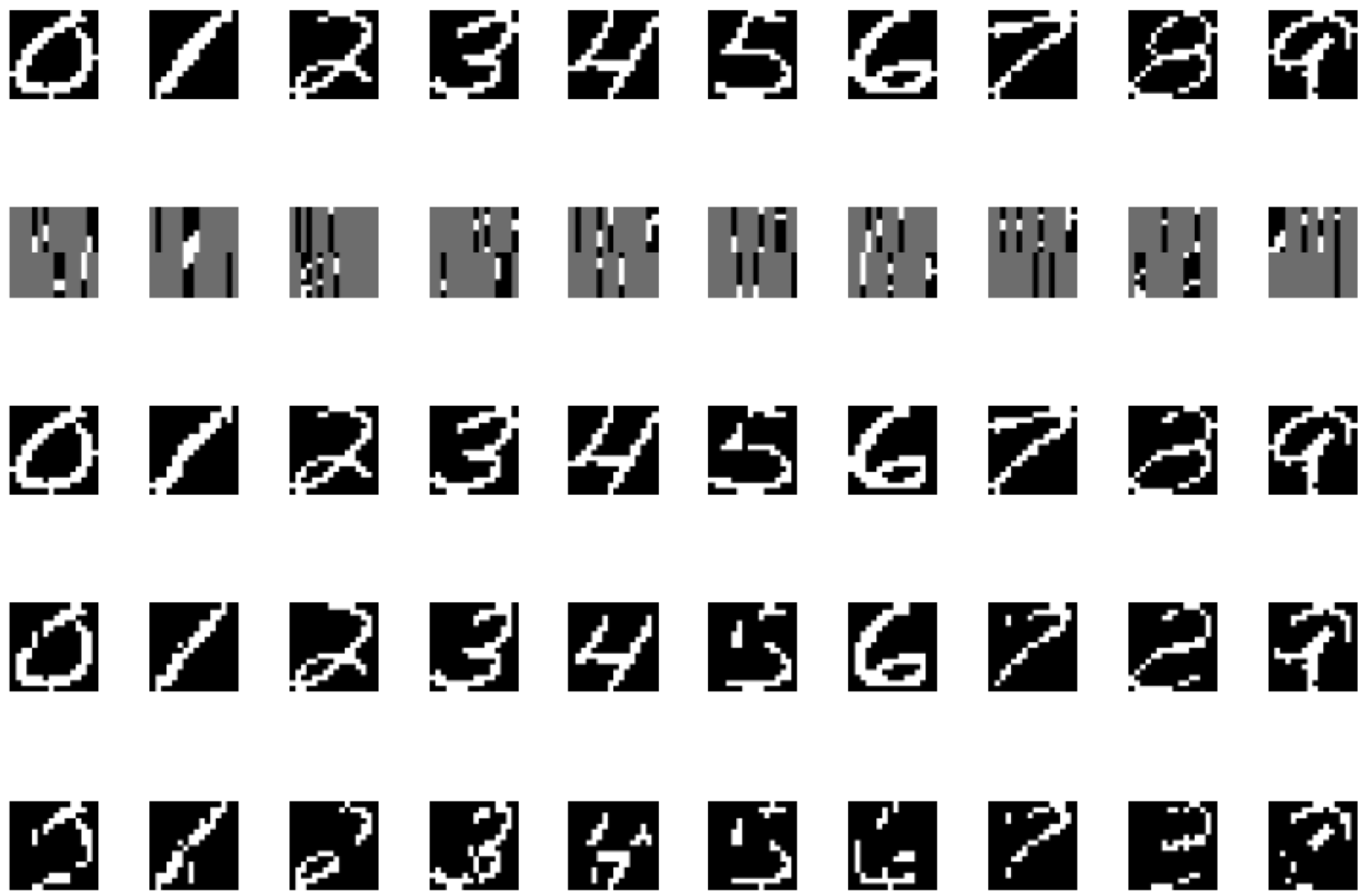Yao, Z.V. Gripon, and M. G. RabbatHigh Performance Computing & Simulation (HPCS), 2014 International Conference on, Blogna, Italy, IEEE, pp. 688 - 692, 07/2014.